# Naive Bayes from scratch

The title summarizes well the subject of this project. Implementation from scratch is the best way to truly understand a model (although intimidating initially). The project helped me learn how to apply data pre-processing and improve my Python programming skills.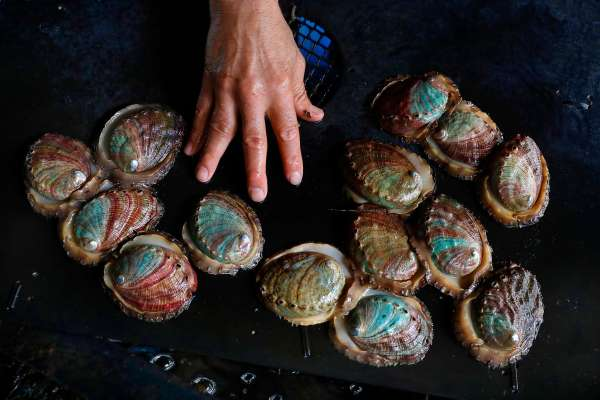This study shows the analysis of Gaussian naive Bayes to predict the abalone age [Y. Han, 2019]. The nature of labeling an abalone being older than 9 years old or not given a set of physical measurements is a valuable task for both farmers and customers. To determine the price of the shellfish, farmers estimate the abalone’s age by cutting the shells and counting the rings through the microscope, a very complex and costly method due to the variation in their size and growth characteristics.

## Naive Bayes Classifier

We will first start with naive Bayes classifier (NBC). Recall, if the features are mutually-independent. Then NBC estimates the prior probabilities of each hypothesis and the probability of each instance.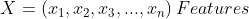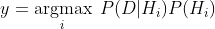Notice, the product can lead to numbers approaching 0 which will cause underflow. The latter term refers to the event when a floating-point data runs out of precision due to extremely tiny numbers. The common work around is to use log-probabilities.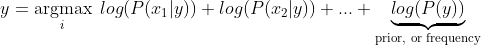We now observe the simple task left ahead. Build the necessary framwork to evaluate the max posterior and the classifier will have accomplished its role.

        # Prob of binary categorical number of occurences/total
for col in cat.columns:
unique = cat[col].value_counts()
unique = unique.apply(lambda x: x/class_count)
self.likelihoods[s][col] = unique

# Prob of binary
for col in bin.columns:
unique = bin[col].value_counts()
unique = unique.apply(lambda x: x / class_count)
self.likelihoods[s][col] = unique

self.priors[s] = class_count / float(num_samples)

    def max_posterior(self, [...]):
posteriors = []
# probability for each class
for i in self._classes:
prior = np.log(self.priors[i])
# sum of all the n feature probabilities
cont_likelihood = np.log(self.normal(i, cont))
cont_likelihood = np.sum(cont_likelihood[~np.isnan(cont_likelihood)])
cat_likelihood = np.sum(np.log(self.categorical_likelihood(i, cat)))
bin_likelihood = np.sum(np.log(self.categorical_likelihood(i, bin)))
likelihood = cont_likelihood + cat_likelihood + bin_likelihood
posterior = prior + likelihood
# posteriors contain the probabilities of the two labels for each instance
posteriors.append(posterior)

return self._classes[np.argmax(posteriors)]


## Data Preprocessing

Data transformation by standardization is applied since it has been shown to speed up convergence via gradient descent [ S. Ioffe and C. Szegedy, 2015] and implicitly balances the contribution of all features. The shellfish abalone rings label with values ranging from 4 to 22 years old was binarized into 1 for all values 9 years old and younger and 0 for all values older than 9 years old. Standardization is also required for proper L2 regularization model. Thus, the data set is the outcome of data cleaning, relevance analysis, data transformation and data reduction.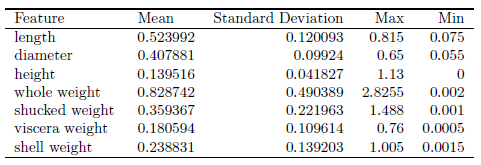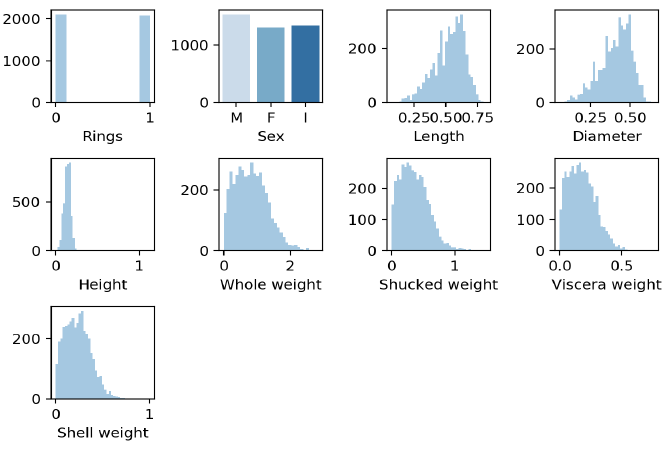## Results

After hyperparameter tuning, Naive Bayes is trained on the entire train split and tested on the unseen test split for all data sets. The model was first validated using 5 fold cross validation; then trained on the train split and evaluated on the test split.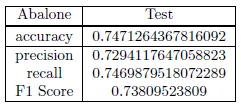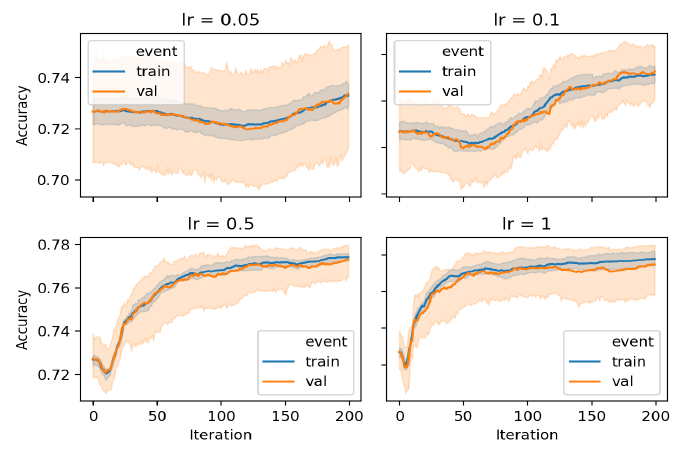# Sources

• S. Ioffe and C. Szegedy, “Batch normalization: Accelerating deep network training by reducing internal covariate shift,” 2015.

• Y. Han, Machine Learning Project - Predict the Age of Abalone. PhD thesis, 06 2019.